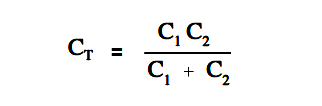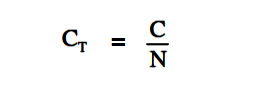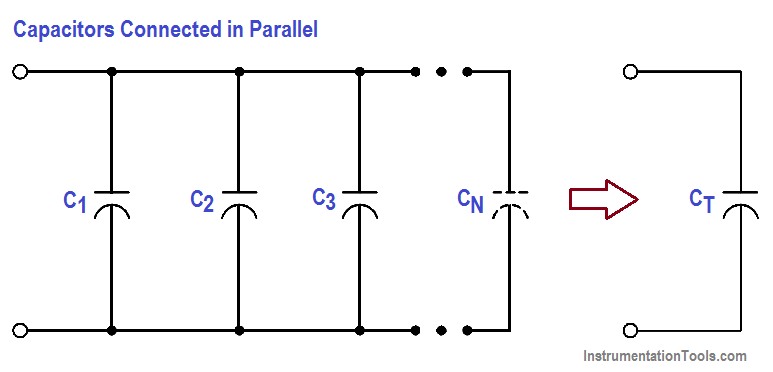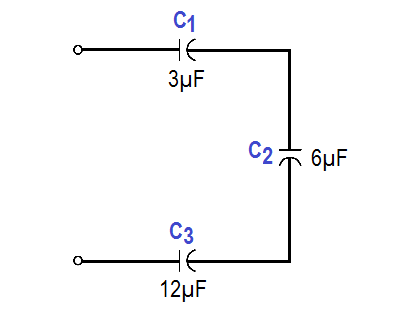# Series and Parallel Capacitors

Capacitors in series are combined like resistors in parallel.Figure 13 : Capacitors Connected in Series

The total capacitance, CT, of capacitors connected in series (Figure 13), is shown in below Equation.When only two capacitors are in series, Above equation may be simplified as shown below.The above Equation is valid when there are only two capacitors in series.

When all the capacitors in series are the same value, the total capacitance can be found by dividing the capacitor’s value by the number of capacitors in series as given in below Equation.where

C = value of any capacitor in series
N = the number of capacitors in series with the same value.

Capacitors in parallel are combined like resistors in series. When capacitors are connected in parallel (Figure 14), the total capacitance, CT , is the sum of the individual capacitances as given in below Equation.

##### CT = C1 + C2 + C3 …….. + CNFigure 14 : Capacitors Connected in Parallel

Example 1:

Find the total capacitance of 3µF, 6µF, and 12µF capacitors connected in series (Figure 15).Figure 15  : Example 1 – Capacitors Connected in Series1/C= 1/3 + 1/6 + 1/12

CT = 12/7 = 1.7 µF

Example 2:

Find the total capacitance and working voltage of two capacitors in series, when both have a value of 150 µF, 120 V (Figure 16).Figure 16 : Example 2 – Capacitors Connected in SeriesCT = 150/2 = 75 µF

Total voltage that can be applied across a group of capacitors in series is equal to the sum of the working voltages of the individual capacitors.

working voltage = 120 V + 120 V = 240 volts

Example 3:

Find the total capacitance of three capacitors in parallel, if the values are 15 µF – 50 V, 10 µF-  100 V, and 3 µF – 150 V (Figure 17). What would be the working voltage?Figure 17 : Example 3 – Capacitors Connected in Parallel

##### CT = C1 + C2 + C3

CT = 15µF + 10µF + 3µF = 28µF

The working voltage of a group of capacitors in parallel is only as high as the lowest working voltage of an individual capacitor. Therefore, the working voltage of this combination is only 50 volts.